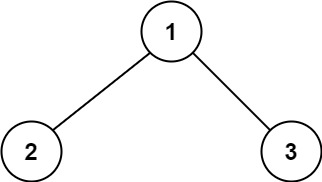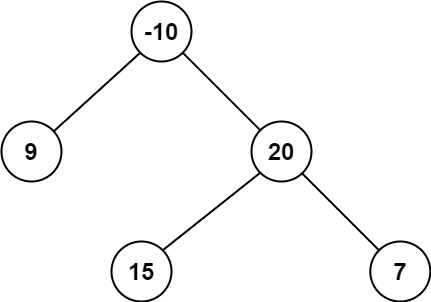# 124. Binary Tree Maximum Path Sum | Leetcode Solution

## 124. Binary Tree Maximum Path Sum | Leetcode Solution

path in a binary tree is a sequence of nodes where each pair of adjacent nodes in the sequence has an edge connecting them. A node can only appear in the sequence at most once. Note that the path does not need to pass through the root.

The path sum of a path is the sum of the node's values in the path.

Given the `root` of a binary tree, return the maximum path sum of any non-empty path.

Example 1:```Input: root = [1,2,3]
Output: 6
Explanation: The optimal path is 2 -> 1 -> 3 with a path sum of 2 + 1 + 3 = 6.
```

Example 2:```Input: root = [-10,9,20,null,null,15,7]
Output: 42
Explanation: The optimal path is 15 -> 20 -> 7 with a path sum of 15 + 20 + 7 = 42.
```

Constraints:

• The number of nodes in the tree is in the range `[1, 3 * 104]`.
• `-1000 <= Node.val <= 1000`
/**
* Definition for a binary tree node.
* struct TreeNode {
*     int val;
*     TreeNode *left;
*     TreeNode *right;
*     TreeNode() : val(0), left(nullptr), right(nullptr) {}
*     TreeNode(int x) : val(x), left(nullptr), right(nullptr) {}
*     TreeNode(int x, TreeNode *left, TreeNode *right) : val(x), left(left), right(right) {}
* };
*/
class Solution {
public:
int ans=INT_MIN;
unordered_map<TreeNode*,int>m;
int helper(TreeNode* root){
if(root==NULL)return 0;
int left=  max(0,helper(root->left));
int right= max(0,helper(root->right));
ans=max(root->val+left+right,ans);
return max(left,right)+root->val;
}

int maxPathSum(TreeNode* root) {
helper(root);
return ans;

}
};